Previous: Th. Corbard's page

## The solar core rotation from LOWL and IRIS or BiSON data

Th.Corbard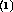, G. Berthomieu, J. Provost, E. Fossat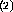(2) Département d'Astrophysique, UNSA, Nice

Abstract

The solar core rotation is investigated by means of a 2D regularized least square inversion. A reliable rotation rate above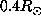is obtained by using the rotational splittings measured between 1994 and 1996 with the data of the LOWL instrument wich can observe both low and intermediate degree p-modes. For sounding the deeper layers, we have alternately added the most recent published splittings of the IRIS and the BiSON network groups. These two sets have been known to be inconsistent at more than a 3-sigma level. The consequences of this difference on the behaviour of the rotation rate inside the solar core, seen by the global inversion, are studied and discussed in this poster.

LOWL data

The LOWL data contain 1102 modes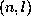with degrees up to l=99 and frequencies lower than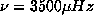. For each mode, individual splittings are given by, at best, three a-coefficients of their expansion on orthogonal polynomials defined by Schou et al. (1994).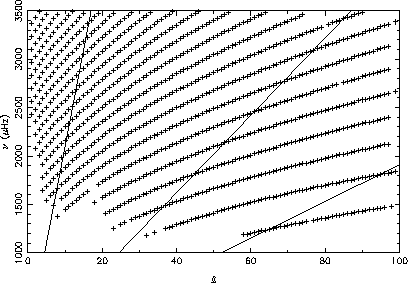This figure is a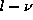diagram showing the modes included in the LOWL 2 years dataset. Solid lines indicate the value of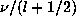that correspond to modes with turning points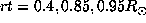from the left to the right.

Measurements of low l degrees rotational splitting frequencies

An observable consequence of the solar rotation is that the azimuthal degeneracy of global modes of oscillation is raised. The rotational splitting is defined by :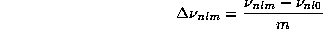where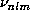denotes the frequency of the mode of degree l, radial order n and azimuthal order m.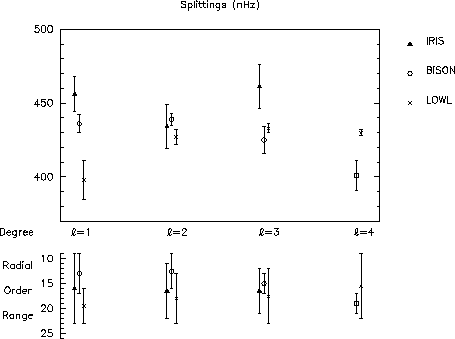This figure gives some recent results obtained by various groups for the low l degree rotational splitting frequencies. The value of the splitting corresponds to an average over the given radial order range assuming that all the splittings for a given degree l are measuring the same quantity. The weighting coefficients used to compute the mean splitting values have been used in the inversion process too.

• IRIS measurements cover a total of 19 months of observation with 4 time series obtained in 1989, 1990, 1991 and 1992 (Gelly et al. 1996). The given splittings are the weighted averages of the results obtained by different methods applied on the same averaged spectra (Lazrek et al. 1996).

• BiSON data have been collected between 05/01/93 and 08/23/94. The plotted values of splittings for each degree l results from a weigthed (by the inverse of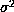) average over n of the splittings obtained for each modeby Chaplin et al. (1996) from the 16-month power spectrum.

• LOWL values have been calculated from the data covering 2 years of observation between 2/26/94 and 2/25/96 (communicated by Steven Tomczyk). The plotted values results from a weighted (by the inverse of) average over n of the a1 coefficients obtained for each mode. In the minimization process, individuals splittings have been built from the given a-coefficients and the weighting coefficients have been taken as the inverse of uncertainties on the individual splittings which have been calculated by assuming them uncorrelated and independent of m for eachand such that they lead at best (in least-squares sense) to the quoted errors given on the a-coefficients. By this way a weigthed average over n and m of these individual splittings leads to the same values and errors as the ones shown on the figure for l=1,2,3,4.

The 2D RLS inversion method

For low rotation the frequency shifts are given by: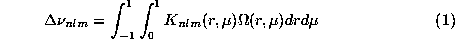where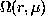is the unknown rotation rate versus depth and latitude,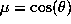,is the colatitude and r the normalized solar radius. The kernels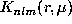depend on the model and the eigenfunctions of the mode which are assumed to be known exactly (e.g. Christensen-Dalsgaard & Berthomieu 1991).

We search for the unknown rotation as a linear combination of piecewise polynomials, projected on a tensorial product of B-spline basis (Corbard et al., 1996). We take into account the observational errors (with standard deviation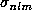) and a regularization term to avoid the large spurious variations of the solution induced by the ill-conditioned inversion problem. Thus we search coefficients that represent the projection of the rotation rate on the chosen B-splines basis by minimizing: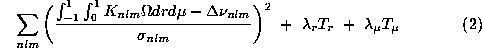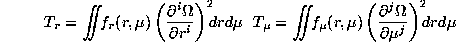The regularization parameters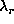and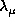and functions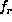and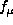are used to define the trade off between the resolution and the propagation of the input errors. We can choose any derivative of the rotation rate. This leads to different behavior of the rotation especially at locations where the observed modes are not enough to constrain the solution. For the results discussed here we choose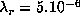,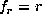, i=2 and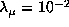,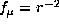, j=1 .

The inversion depends on the number of piecewise polynomials, on the order of the spline functions and on the distribution of the break points of these polynomials. In what follows, the distribution of these points along the radius has been chosen according to the density of the turning points of the considered p-modes for a given dataset.

A complete description of the method and the choice of the parameters used for inverting LOWL data can be found in Corbard et al. (1996)

Results and discussion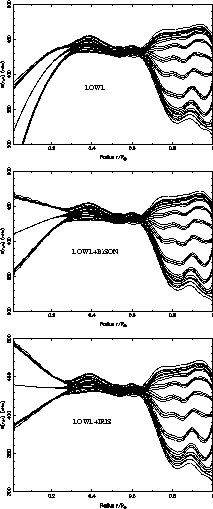The above figures show the inferred rotation rate for ten latitudes (bold curves for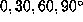) obtained by inverting
LOWL data, LOWL+IRIS data and LOWL+BiSON data. The 3 solutions differ only under 0.2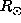and show no latitudinal dependencies below this depth. The solution obtained between 0.4 and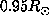is discussed in Corbard et al. (1996).LOWL data are compatible within 1error bars with a core that rotate slower than the radiative interior.Solutions with IRIS and BiSON data are compatible and show that an extension to the solar core of the rather uniform rotation found in the outer radiative interior could explain the observed splittings.

The difference between LOWL and IRIS or BiSON data (especially for l=1) is sufficient to produce solutions that are not compatible. This is not surprising since these depths are intrinsically not well constrained so that little variation in the data could produce important variation in the solution. Nevertheless all the available observations argue against theories for angular momentum transport which predict a rotation rate at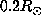very higher than the equatorial rotation rate.

Although the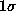error bars shown on the figure are large below, we can not insure that the true rotation rate lies inside these error bars. In fact these bars represent the uncertainties on a weighted averaged of the rotation rate. In an attempt to have a best interpretation of our results we must have a look at the weighting function given by the so-called averaging kernels.

Averaging kernels

The inferred rotation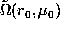at a point (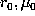) is obtained as a linear combination of the data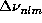. Thus from Eq.(1) it follows that averaging kernels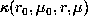, which are a linear combination of rotational kernels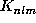, exist such that: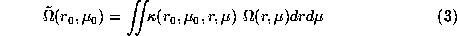The radial and latitudinal full widths at mid height (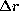and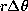) of these kernels provide an estimation of the spatial resolution of the inversion at a point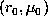. It increases with lower regularizing term, at the expense of larger errors on the solution.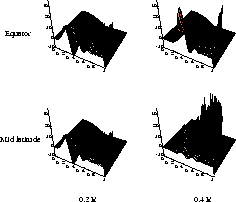These 3D figures show averaging kernels obtained at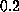andat the equator and mid latitude.

The radial and latitudinal resolution reached atat the equator are respectively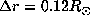and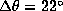. Nevertheless, we obtain a lot of additional peaks localized at the surface. Generally, a well peaked kernel can be obtained only if a large amount of cancelations eliminates the dominant contribution of the rotational kernels near the surface. In this inversion the cancelation is not completely done showing that a better inversion should require observation of high degree modes which are able to give informations about zones near the solar surface. We note however that the peaks shown near the surface remain unresolved with the grid used for the plot and that their contribution to the whole kernel is very small.

Although the two kernels atare well localized, the two ones atare not distinguishable and are both localized at the equator and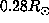. This result shows that our inversion is not able to detect a latitudinal dependency of the rotation rate even if one exists at. The solution obtained at all latitudes below 0.3is an estimation of the rotation rate in the equatorial plane. A more important problem arise from the fact that the averaging kernels calculated at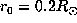and below this depth are still localized between 0.25 and 0.28. This indicates that the true rotation rate at a target location below 0.2contributes only for a few part in the calculation of the inferred rotation rate at this location.

Conclusion

The aim of this work was to test the ability of our 2D inversion code of making inferences about the rotation of the solar core by using the available data concerning the low degree p-modes.

The solutions we obtain show that the different values given by observers for the low l splittings lead to different behaviours of the inferred rotation rate in the core. Therefore the inversion is sensitive to the actual observational differences. Nevertheless the shape of the averaging kernels corresponding to solar core layers makes the interpretation of results in term of the true rotation rate very difficult. For example, the averaging kernel calculated atpresents important oscillations up to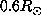. Therefore the interpretation of the value of the rotation rate obtained atdepends strongly on the behavior of the rotation rate in the radiative interior. We note however that the integral of the averaging kernel between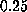andis null. Thus, if we suppose that the rotation rate is constant in the radiative interior and down to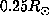, we can interpret the value obtained atas a weighted averaged of the true rotation rate between 0.1 andthe weighting function being the corresponding part of the averaging kernel. Nevertheless although the solid rotation found betweenandis thought to be reliable, its extension down to 0.25is more speculative and some little gradients in these zones can modify significantly the interpretation of the value of the rotation rate found atand deeper.

A better understanding of the rotation of the core should require a better agreement between the different analysis of the different observations. However, this work shows some limits in the utilization of a 2D RLS code to probe the rotation of the core. Some other global inversion techniques as SOLA should be helpful by searching explicitly averaging kernels without any negative part. Such kernels, even larger than those obtained in this work should be useful to complete the interpretation of our results in the core.

Chaplin W.J., Elsworth Y., Howe R., 1996, Solar core rotation: low-degree solar p-mode rotational splitting results from BISON, MNRAS, submitted

Christensen-Dalsgaard J., Berthomieu G., 1991, Theory of Solar Oscillations. In: Cox A.N., Livingstone W.C., Matthews M.S. (eds) Solar Interior and Atmosphere. Univ. of Arizona Press, Tucson, p. 401

Corbard T., Berthomieu G., Provost J. and Morel P., 1996, The solar rotation rate from LOWL data: A 2D regularized least-squares inversion using B-splines, 1996, to be submitted to A&A

Gelly B., Fierry-Fraillon D. et al., 1996, submitted to A&A

Gizon L., Fossat E., Lazrek M. et al., 1996, New IRIS constraints on the solar core rotation, submitted to A&A Letters

Lazrek M., Pantel A., Fossat E., et al., 1996, Is the solar core rotating faster or slower the the envelope?, Sol. Phys., 166, 1

Schou J., Christensen-Dalsgaard J., Thompson M.J., 1994, On comparing helioseismic two-dimensional inversion methods, ApJ 433, 389

Tomczyk S., Schou J., Thompson M.J., 1995b, Measurement of the Rotation Rate in the Deep Solar Interior, ApJ 448, L57Thierry Corbard
E-mail: corbard@obs-nice.frFax: (+33) 04 92 00 31 21
Dernières modifications: 16 Octobre 1996